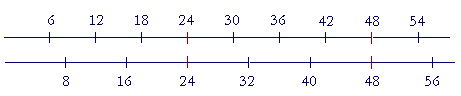S k i l l
i n
A R I T H M E T I C

Lesson 23

# LOWEST COMMON MULTIPLE

## HOW TO COMPARE FRACTIONS

WE WILL SEE that to add fractions, or to compare fractions that have different denominators, we must construct a common denominator. What denominator should we choose? We should choose the lowest common multiple of the original denominators. The student therefore must be clear as to what that means.

Here are the first few multiples of 6:

6,  12,  18,  24,  30.

And here are the first few multiples of 8:

8,  16,  24,  32,  40.

24 is a common multiple of 6 and 8.  It is their lowest common multiple, which we abbreviate as the LCM.The LCM is the first time that the multiples of 6 meet the multiples of 8.

 1. How can we find the lowest common multiple of two numbers? Go through the multiples of the larger numberuntil you come to a multiple of the smaller number.

Example 1.   Find the LCM of 9 and 12.

Solution.   Go through the multiples of 12 until you come to a multiple of 9.

12,  24,  36.

36 is the first multiple of 12 that is also a multiple of 9.  36 is their LCM.

Example 2.   Find the LCM of 2 and 8.8 itself is their LCM.

When the larger number is itself a multiple of the smaller number, then the larger number itself is their LCM.

Example 3.   Find the LCM of 5 and 20.

Solution.   20 is their LCM.

Now the product of two numbers will always be a common multiple.  The product of 6 and 4, for example, is 24, and 24 is a common multiple -- but it is not their lowest common multiple.  Their lowest common multiple is 12.

 2. When is the LCM of two numbers simply their product? When they have no common divisors except 1.

Compare Lesson 22, Question 4.

Example 4.   What is the LCM of 10 and 27?

Answer.  10 and 27 have no common divisors except 1.  Therefore their LCM is 10 × 27 = 270.

(1 is a common divisor of every pair of numbers, but some pairs have 1 as their only common divisor.  10 and 27 are such a pair.)

Example 5.   What is the LCM of 8 and 12?

Answer.  24.  Their LCM is not 8 × 12, because 8 and 12 have common divisors besides 1;  for example, 4.

(To find the LCM from prime factors, see Lesson 33.)

Comparing fractions

In Lesson 20 we saw how to compare fractions that have equal numerators or equal denominators.  We will now see how to compare any two fractions.

 3. How can we compare fractions when the numerators and denominators are different?Change them to equivalent fractions that will have equal denominators. As the common denominator, choose the LCM of the original denominators. Then the larger the numerator, the larger the fraction.
 Example 6.   Which is larger, 12 or 38 ?

Answer.  Make a common denominator.  Choose the LCM of 2 and 8 -- which is 8 itself.  Example 2, above.

 We will change 12 to an equivalent fraction with denominator 8:
 12 = 48 .
 The denominators are now the same, and we can compare 48 with 38 .

We see:

 48 is larger than 38 .

That is,

 12 is larger than 38 .

 Example 7.   Which is larger, 34 or 2532 ?

Answer.  Again, we will make the denominators the same, and then compare the numerators.  As a common denominator, we will choose the LCM of 4 and 32, which is 32 itself.

 We will express 34 with denominator 32. On multiplying both

terms by 8,

 34 = 2432 .
 We are now comparing 34 , or
 2432 with 2532 .
 25 is larger than 24; therefore 2532 is larger than 34 .

 Example 8.   Which is larger, 56 or 79 ?

Answer.  As a common denominator, choose the LCM of 6 and 9.

 56 = 1518 , 79 = 1418 .
 To change 56 , we multiplied both terms by 3.  To change 79 , we

multiplied both terms by 2.

We choose a common multiple of the denominators because we change denominators by multiplying themNow, 15 is larger than 14. Therefore, 56 is larger than 79 .

Adding fractions (as we will see in Lesson 25) involves the same technique as comparing them, because the denominators -- the units -- must be the same.  For example,

 56 + 79 = 1518 + 1418 = 2918 .

In the next Section, Question 4, we will see how to compare fractions by cross-multiplying.

At this point, please "turn" the page and do some Problems.

or

Continue on to the next Section.